# Looping – js1k 2018 post mortem

## blog

For this year’s js1k I wanted to build a simple ray-tracer to see both how much could I fit in 1k and the performance of js.
I started by adding a very simple (trivial) camera implementation and adding a sphere primitive: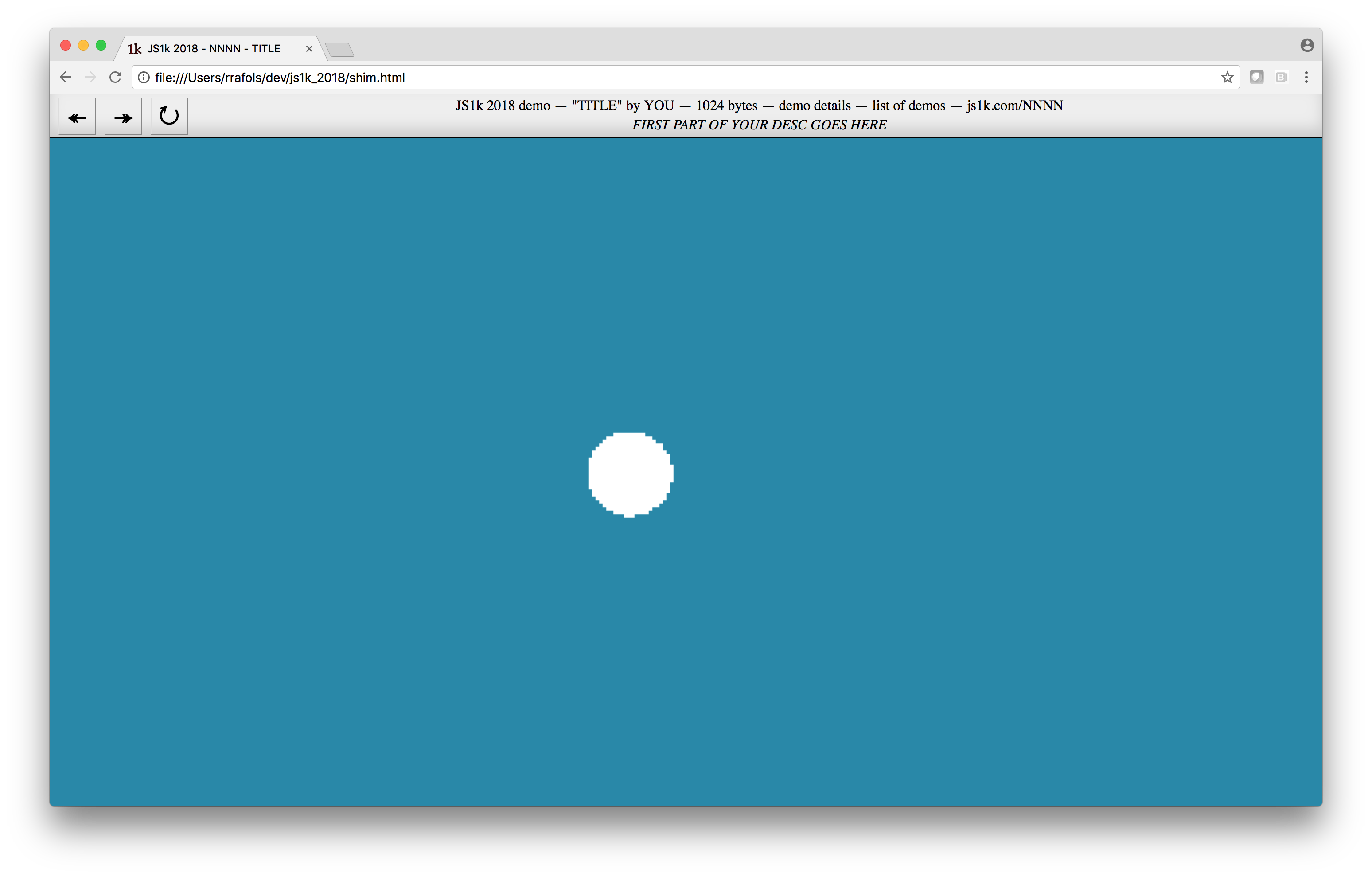Results were not mind blowing but, hey, that was a start. Code was pretty simple:

``````//w = canvas width
//h = canvas height
//F = pixels skipped. At F=1 we would compute the real value each pixel, at 2, we would compute at every 2 pixels, and so on..
// where the camera is and where the camera is looking
origin=[0,0,-1000]
dst  =[0,0,1000]
fov = 90
...
// compute direction vector and horizontal & vertical vectors (hv & vv respectively)
cd=[dst-origin,
dst-origin,
dst-origin]
u(cd)
alpha = Math.PI*fov/360.0
focaldist = w * Math.cos(alpha)/(2*Math.sin(alpha))
cv=[origin+cd*focaldist,
origin+cd*focaldist,
origin+cd*focaldist]
hv=[-cd,0,cd]
vv=x(hv, cd)
// compute the view vector
v=[cv-vv*h2-hv*w2-origin,
cv-vv*h2-hv*w2-origin,
cv-vv*h2-hv*w2-origin]
for(i=0;i&lt;h;i+=F) {
vl=[v, v, v];
for(j=0;j&lt;w;j+=F) {
d = [vl,vl,vl]
u(d)
os = it(origin, d)
if(os != -1) {
c.fillStyle='#fff'
} else {
c.fillStyle='#28A'
}
c.fillRect(j, i, F, F)
// we add the computed camera horizontal vector for each column
vl += hv * F
vl += hv * F
vl += hv * F
}
// we add the computed camera vertical vector for each row
v += vv * F
v += vv * F
v += vv * F
}
``````

Functions u & x are two small utilities to calculate the unit vector and cross product:

``````function u(v) {
m = Math.sqrt(v * v + v * v + v * v)
v/=m
v/=m
v/=m
}
function x(u,v) {
k=[]
k=u*v - u*v
k=-(u*v - u*v)
k=u*v - u*v
return k
}
``````

and the intersection code was straightforward:

``````function it(o, d) {
os = -1
maxt = 100000000.0
for(k=0;k0) {
t=tca-Math.sqrt(thc);
}
}
if(t&lt;maxt) {
maxt = t;
os = k
}
}
return [os, maxt]
}
``````

Shading was trivial as well (if we can even call it shading):

``````os = it(origin, d)
if(os != -1) {
c.fillStyle='#fff'
} else {
c.fillStyle='#28A'
}
``````

Draw it white if there is an intersection or background-blueish if it isn’t.
Now that we get the basics in place, let’s add more spheres, colors and some shading:

``````os = it(origin, d)
if(os != -1) {
// point of intersection: origin + direction ray * t
ol=[origin + d * os,
origin + d * os,
origin + d * os]
// intersection point normal (point - sphere center)
ir=sphereList[os*S + 5]
n=[(ol - sphereList[os*S]) * ir,
(ol - sphereList[os*S+1]) * ir,
(ol - sphereList[os*S+2]) * ir,
]
u(n)
// light position
ll=[0,-1000,0]
// intersection point -&gt; light direction vector
rl=[ll - ol,
ll - ol,
ll - ol]
u(rl)
// sphere color
r=sphereList[os*S + 5]
g=sphereList[os*S + 6]
b=sphereList[os*S + 7]
// color factor = dot product of normal and light direction vector
al=n*rl + n*rl + n*rl
if(al&gt;0.0){
r*=al
g*=al
b*=al
} else {
r=0
g=0
b=0
}
r=(r*16)|0
g=(g*16)|0
b=(b*16)|0
c.fillStyle='#'+hexmap[r]+hexmap[g]+hexmap[b];
} else {
c.fillStyle='#28A'
}
``````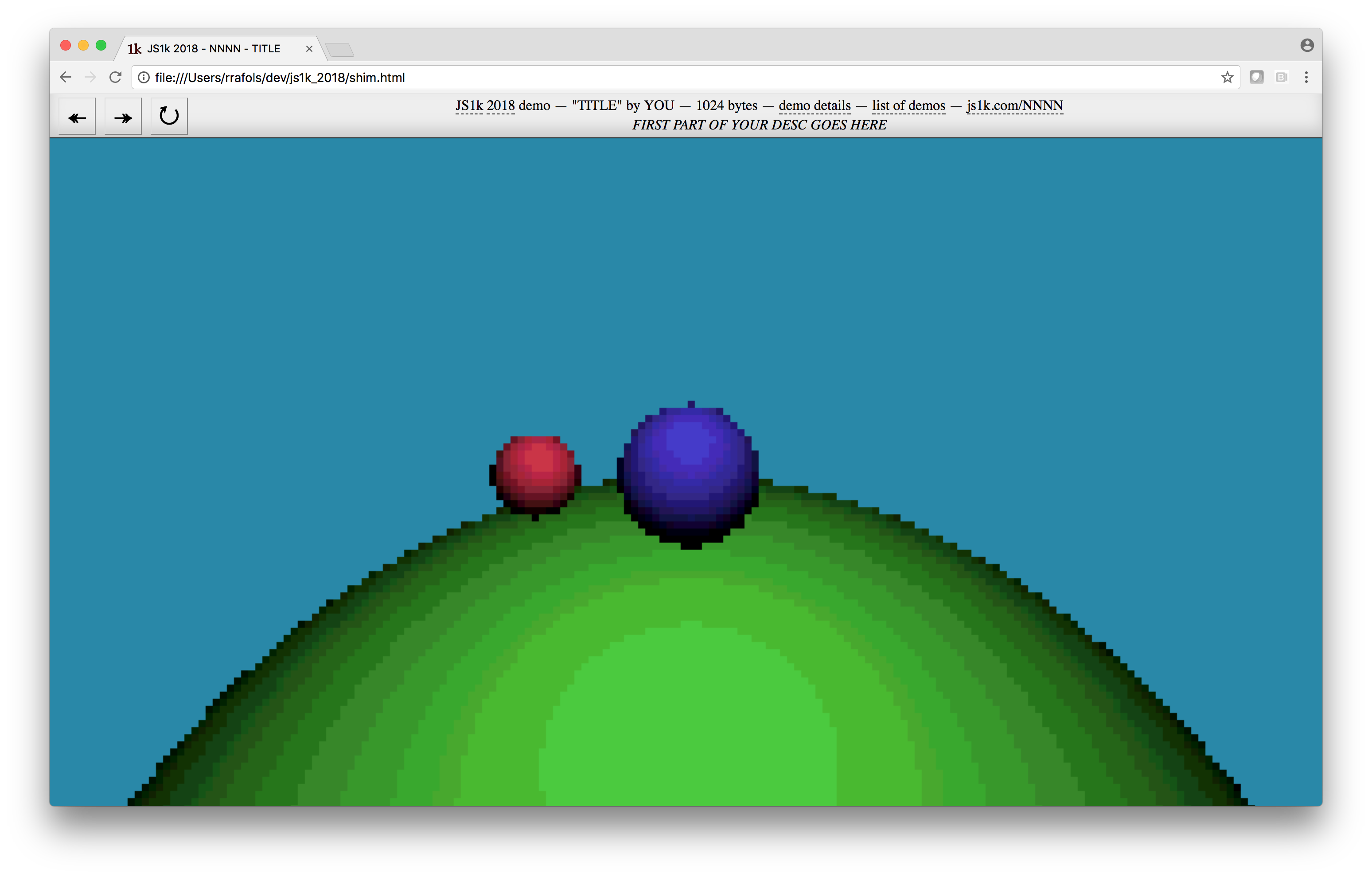So, let’s add a specular component to the light:

``````// compute the reflection of the ray
al=2*(n*d+n*d+n*d)
rx=[d - al * n,
d - al * n,
d - al * n
]
// and the dot product with the light vector
ls=rx*rl+
rx*rl+
rx*rl
// the higher the exponent, more focused will be the specular:
ls=ls0.0){
r=r*al+ls
g=g*al+ls
b=b*al+ls
}
``````

And here the result: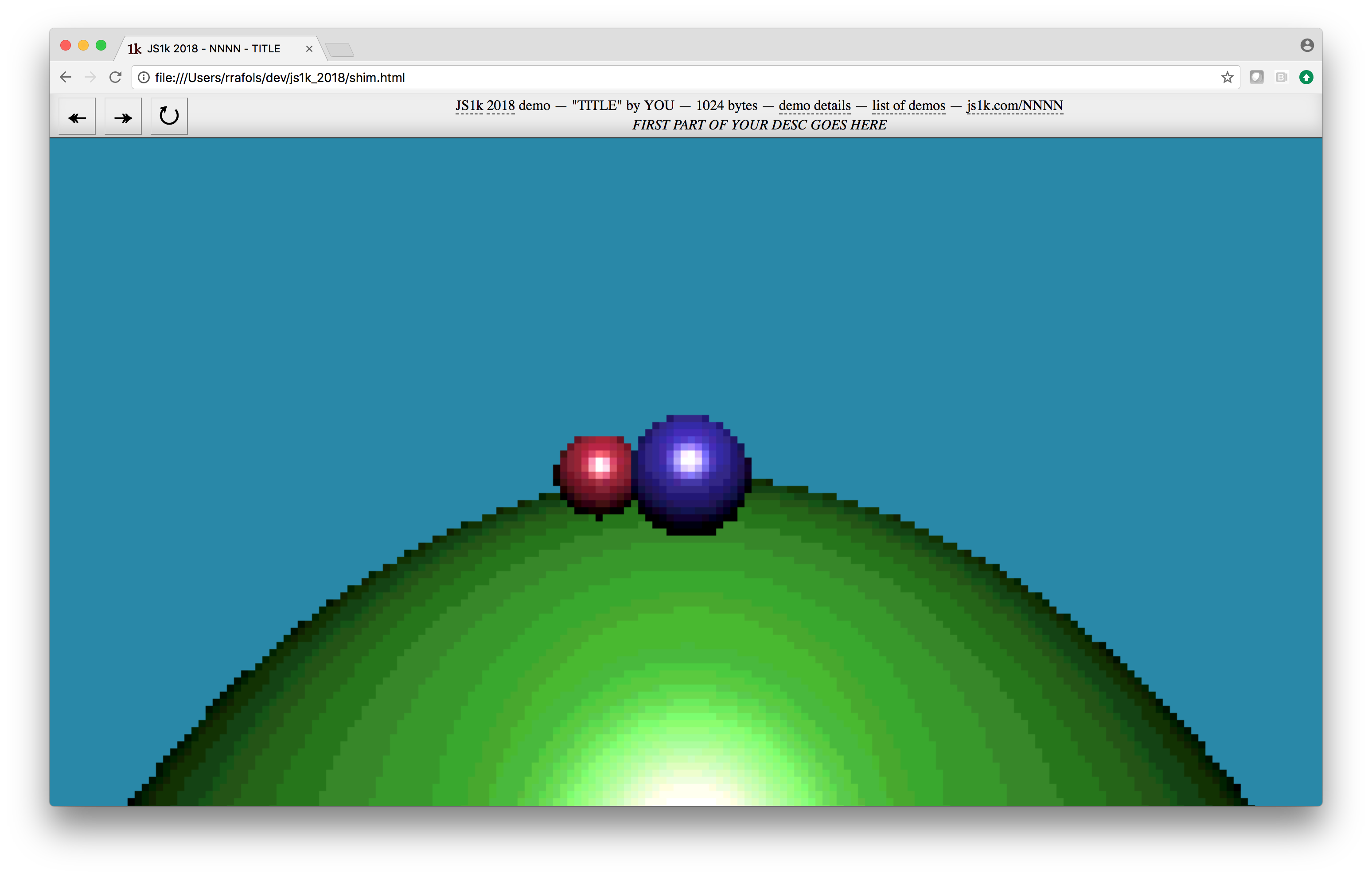We can also play with the specular exponent. Below the differences between 20, 8 and 2: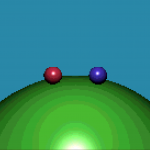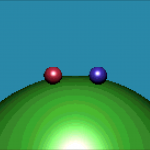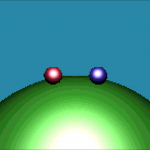So far, things are quite simple, but what would be a raytracer without shadows? Let’s also add some shadows!
As we have one single light, it is quite straightforward, before shading each pixel, trace a ray from the intersection point to the light position. If there is any positive intersection of an object it means that point is occluded and shouldn’t be shadowed. As we already have the function to calculate intersections written, it also very simple:

``````// compute the 't' value of the equation. This will be used as the maximum value for the 't'. Any object further than that will not occlude the light
tl=(ll - ol)/rl
sh=it(ol, rl, tl, os)
// if not intersection, let's shade the pixel normally
if(sh==-1){
...
...
} else {
// pixel is shadowed, apply only ambient color
}
``````

Result starts to look pretty decent: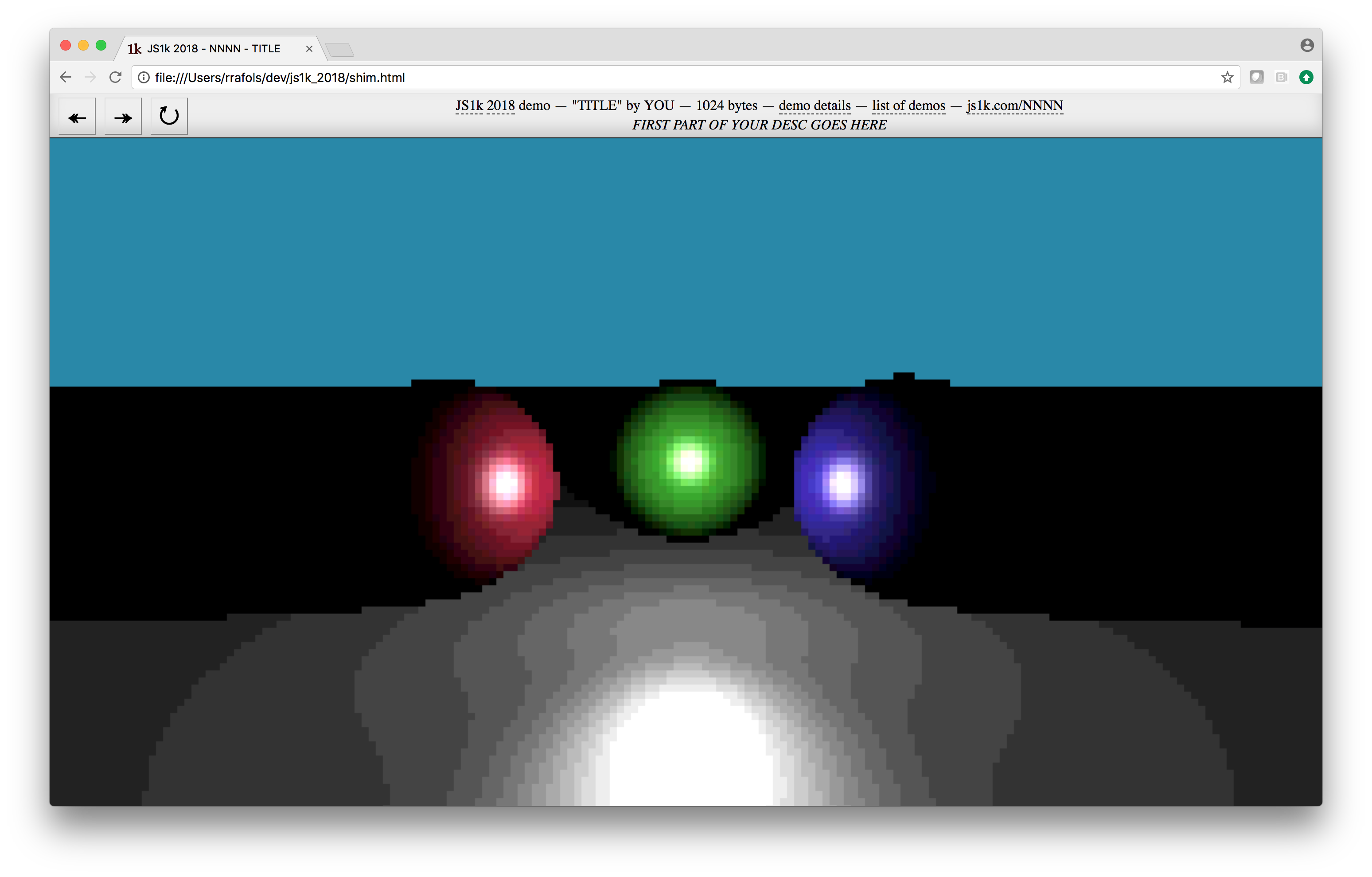I’ve also added another primitive: a horizontal plane:

``````if(sl[k*S+7] == 0) {
...
// sphere intersection
...
} else {
vd=sl[k * S + 0] * d + sl[k * S + 1] * d + sl[k * S + 2] * d;
vo=-sl[k * S + 0] * o + sl[k * S + 1] * o + sl[k * S + 2] * o + sl[k * S + 3];
t=vo/vd
}
``````

``````sl = [1000, 500, 2000, 250000, 0.8, 0.2, 0.3, 0,
-1000, 500, 2000, 250000, 0.3, 0.2, 0.8, 0,
0, 500, 3000, 250000, 0.3, 0.8, 0.2, 0,
0, 1, 0, 1000, 0.6, 0.6, 0.6, 1
]
``````

Entries in this array have the following meaning:

``````// x
// y
// z
// red
// green
// blue
// object type (0 - sphere, 1 - horizontal plane)
``````

Normal has to be computed in a different way depending on the primitive type. In the case of the plane I was planning to do a checkerboard, but to save some space I ended up creating stripes:

``````r=sl[os*S + 4]
g=sl[os*S + 5]
b=sl[os*S + 6]
// normal reset to vertical vector
n=[0,-1,0]
if(sl[os*S+7]==0) {
// if we intersect a sphere, let's recalculate the normal
n=[(ol - sl[os*S]),
(ol - sl[os*S+1]),
(ol - sl[os*S+2])
]
u(n)
} else {
//tweak color depending on distance - the 1000 was chosen in the most scientific way possible - keep playing with the value until it feels right
cl=(ol/1000|0)%2
r=1
b=0
if(cl==0) {
g=1
} else {
g=0
}
}
``````

Result was the following: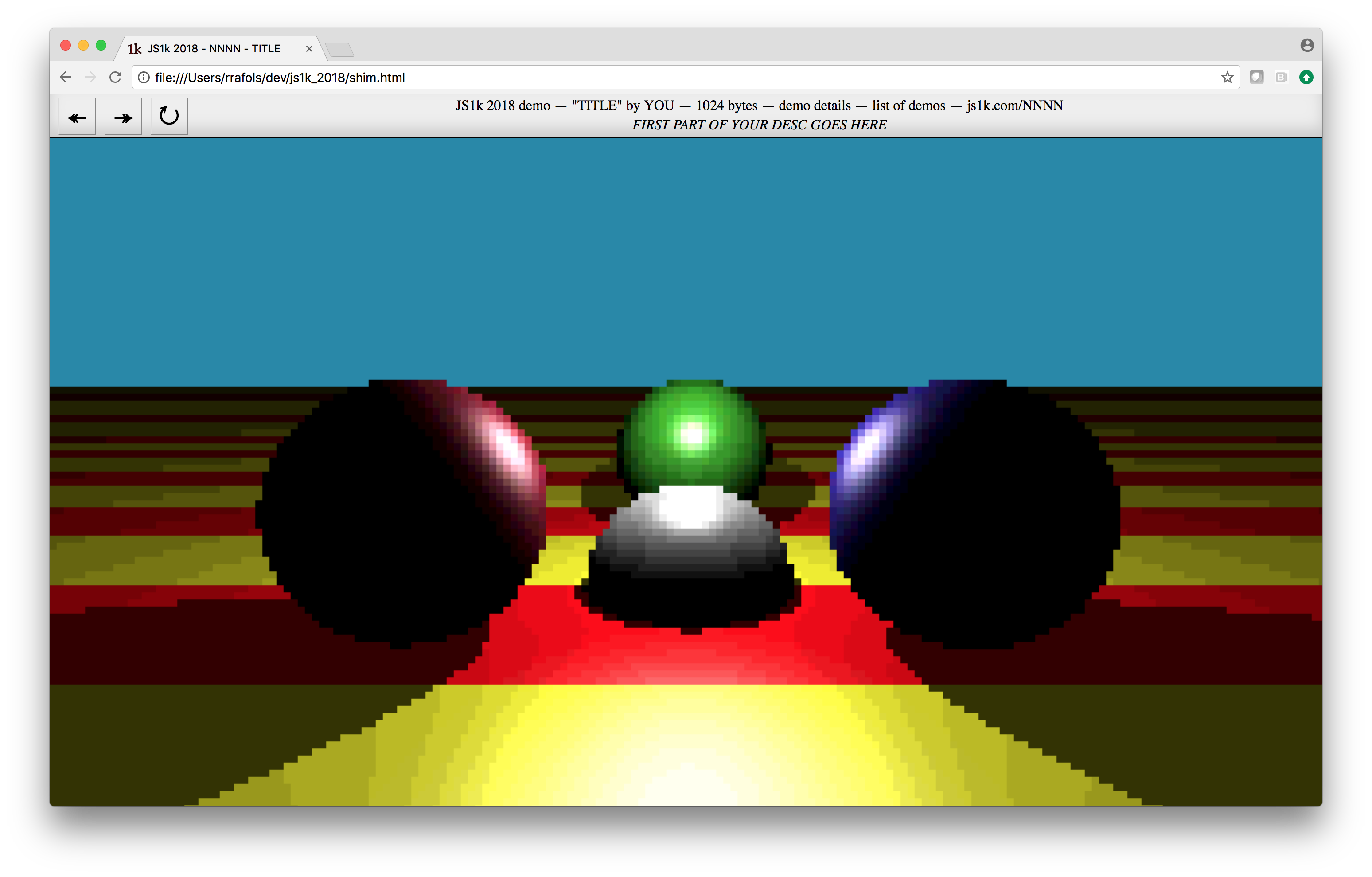We’re still missing one key feature of raytracers, reflections! In order to add reflections, we need to create a function that calculates the intersection & computes the resulting color. We’ll call it recursively:

``````// if reflection index is smaller than 3, compute a reflected ray color & merge it with current color (70% + 30%)
// we limit the amount of reflections to 3 levels
if(rc &lt; 3) {
rxc = cil(ol, rx, rc+1)
r=r*0.7+rxc*0.3
g=g*0.7+rxc*0.3
b=b*0.7+rxc*0.3
}
``````

By adding reflections, looks slightly better: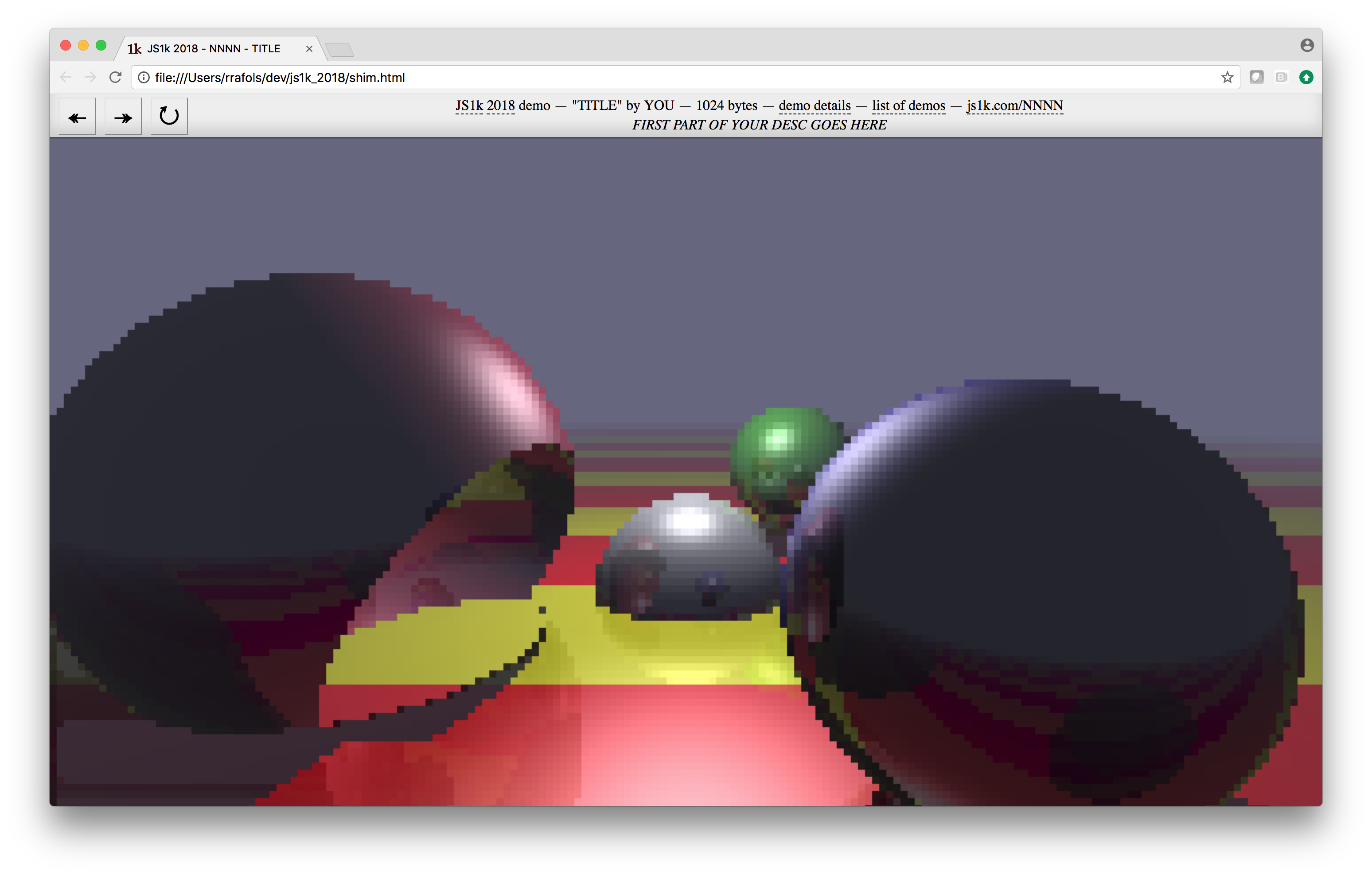Also, as you could see in this last screenshot, there was an small intent of CSG (Constructive Solid Geometry). I added a sphere that was ‘substracting’ from other primitives.
At the end, I managed to fix the CSG intersection code. In order to make it work we’ve to calculate both the minimum and the maximum ‘t’ of the ray intersection and work out all the different options:

``````---- direction ray
* intersection of original object
# intersection of the subtracting object

trivial case, normal intersection
1)---------*----*----#---#-------
trivial case, normal intersection
2)---------#----#----*---*-------
trivial case, normal intersection
3)---------*----#----#---*-------
trivial case, normal intersection
4)---------*----#----*---#-------
trivial case, no intersection (subtracting object is wrapping the whole object)
5)---------#----*----*---#-------
intersection would be the exit point of subtracting object (second #)
6)---------#----*----#---*-------
``````

And the updated intersection code. Used a ‘magic’ code -2 to detect we’re checking the CSG intersection: (Kids don’t do that at home!)

``````var csi=0
if(z!=-2 &&t!=t2){
var csg=it(o,d,maxt,-2)
if(csg==5) {
if(csg &lt; t && csg &gt; t && csg &lt; t2) {
t=csg
csi = 1
}  else if(csg &lt; t && csg &gt; t2) {
t = maxt
}
}
}
``````

``````if(sl[k*S+7] == 0) {
// sphere intersection
} else if(sl[k*S+7] == 1) {
// plane intersection
} else {
// cylinder
var rd2=1.0-d*d
var b=sl[k*S + 3]*rd2
var b2=d*(o-sl[k*S + 2]) - d*(o-sl[k*S + 0])
b-=b2*b2
if(b&gt;0) {
b2 = Math.sqrt(b)
b=d*(o-sl[k*S + 0]) + d*(o-sl[k*S + 2])
t=-(b+b2)/rd2
t2=-(b-b2)/rd2
}
}
``````

And here is the result: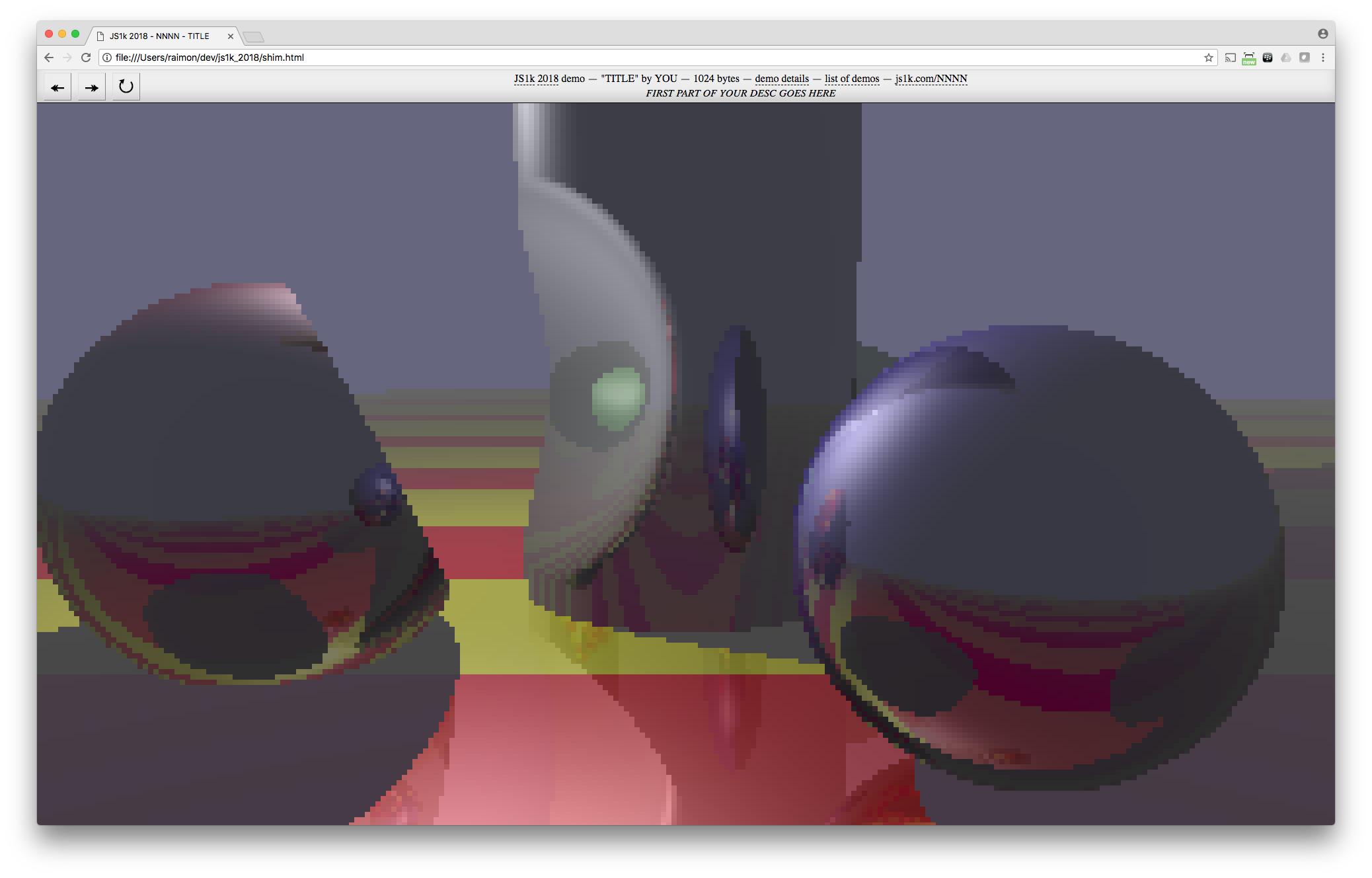Unfortunately, after using Uglify (https://github.com/mishoo/UglifyJS) and RegPack (http://siorki.github.io/regPack.html) the resulting size was over 1600 bytes so I had to start a heavy optimization to get it under 1024. I removed the CSG intersection code :(, simplified the RGB filling color to:

``````r=(col*255)|0
g=(col*255)|0
b=(col*255)|0
c.fillStyle='rgba('+r+','+g+','+b+',1)'
c.fillRect(j, i, F, F)
``````

instead of using the hexmap and 16 different shades per component – I kind of liked it the pixelated/oldschool effect, but it was slightly shorter & definitely faster.
After some time optimizing and doing some sacrifices I managed to get it under 1024. It was a pity I couldn’t spend any more time on it as I would really tried to squeeze the CSG code in! 😉
Here is the final source code:

``````w=window.innerWidth
K=500
J=K*K
E=1000
D=1500
G=0
l=n=[]
O = [0, K, 0, J*2, 1, 0, 0, 0,
0, K, 0, J, 0, 0, 1, 0,
0, K, 0, J, 0, 1, 0, 0,
0, E, E*2, J, 1, 1, 1, 2,
0, 1, 0, E, 1, 0, 0, 1]

// dot product
dp = (u,v) =&gt; u*v+u*v+u*v

// unit vector
u = v =&gt; {
m = Math.sqrt(dp(v,v))
v/=m
v/=m
v/=m
}

it = (o, d, T) =&gt; {
u(d)
s = -1
t = T
for(z=0;z&lt;40;z+=8){
t=(-O[z] * o + O[z + 1] * o + O[z + 2] * o + O[z + 3])/(O[z] * d + O[z + 1] * d + O[z + 2] * d)
if(O[z+7] == 0) {
e=[O[z] - o,
O[z + 1] - o,
O[z + 2] - o]
p=dp(e,d)
t=p-Math.sqrt(O[z+3]-dp(e,e)+p*p)
}
if(O[z+7] == 2) {
p=1-d*d
q=d*(o-O[z + 2]) - d*(o-O[z])
t=-(d*(o-O[z]) + d*(o-O[z + 2])+Math.sqrt(O[z + 3]*p-q*q))/p
}
if(t&gt;.1&&t&lt;T) {
T = t
s = z
}
}
return [s, T]
}

cil = (o,d) =&gt; {
var r=[.4,.4,.5]
I = it(o, d, E*E)
if(I&gt;=0) {
y=I
for(k=0;k&lt;3;k++) {
o[k]=o[k] + d[k] * I
n[k]=o[k] - O[y+k]
l[k]=O[2-k]-o[k]-D*k
}
if(O[y+7]==1) {
n=[0,-1,0]
O[y + 5]=(o/E|0)%2
}
if(O[y+7]==2) n = 0
u(n)
H=it(o, l, E*E)
A=2*dp(n,d)
rx=[d - A * n, d - A * n, d - A * n]
A=L=0
if(H&lt;0) {
L=dp(rx,l)
L=L&lt;0?0:Math.pow(L,9)
A=dp(n,l)
}
for(k=0;k&lt;3;k++) r[k]=O[y+4+k]*A+L
R = cil(o, rx)
for(k=0;k&lt;3;k++) r[k]=(r[k] + R[k])/2
}
return r
}

setInterval(() =&gt; {
//animation
for(i=2;i&gt;=0;i--) {
O[i*8] = l = D*Math.sin(G + i*2)
O[i*8 + 2] = l = D*Math.cos(G + i*2) + D;
}
//render
for(;i&lt;window.innerHeight;i+=6) {
for(j=0;j&lt;w;j+=6) {
x=cil([0,0,-D*2], [w/2-j, i-w/6, D-w/6])
c.fillStyle='rgb('+[x*255|0,x*255|0,x*255|0]+')'
c.fillRect(j, i, 6, 6)
}
}
G+=.02
}, 10)
``````

and the result can be checked here: https://js1k.com/2018-coins/demo/3101